3 May 2017

## ggplot2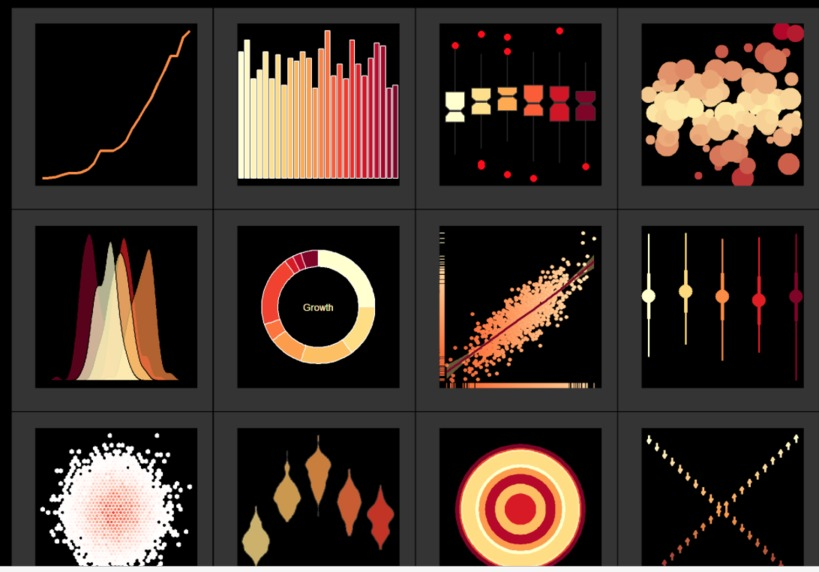## Learning objectives

By the end you should be able to:

• Understand the basic grammar of ggplot2 (data, geoms, aesthetics, facets).
• Make quick exploratory plots of your multidimensional data.
• Know how to find help on ggplot2 when you run into problems.

XX ADD GROUP aes for geom_line

## Before, there was ggplot1

Released in 2005 until 2008 by Hadley Wickham.

If the pipe ( %>% in 2014) had been invented before, ggplot2 would have never existed Hadley Wickham

### Original syntax

# devtools::install_github("hadley/ggplot1")
p <- ggplot(mtcars, list(x = mpg, y = wt))
# need temp p object to avoid too many ()'s
scbrewer(ggpoint(p, list(colour = gear)))

### with the pipe

# devtools::install_github("hadley/ggplot1")
library(ggplot1)
mtcars %>%
ggplot(list(x = mpg, y = wt)) %>%
ggpoint(list(colour = gear)) %>%
scbrewer()

### ggplot2

library(ggplot2)
mtcars %>%
ggplot(aes(x = mpg, y = wt)) +
geom_point(aes(colour = as.factor(gear))) +
scale_color_brewer("gear", type = "qual")

### Issue

Introduced a break in the workflow from %>% to +

## What is ggplot2

### released in 2007

• ggplot2 stands for grammar of graphics plot version 2
• Inspired by Leland Wilkinsons work on the grammar of graphics in 2005.
• The idea is to split a graph into layers: for example axis, curve(s), labels.
• 3 main elements are necessary: data, aesthetics and at least one geometry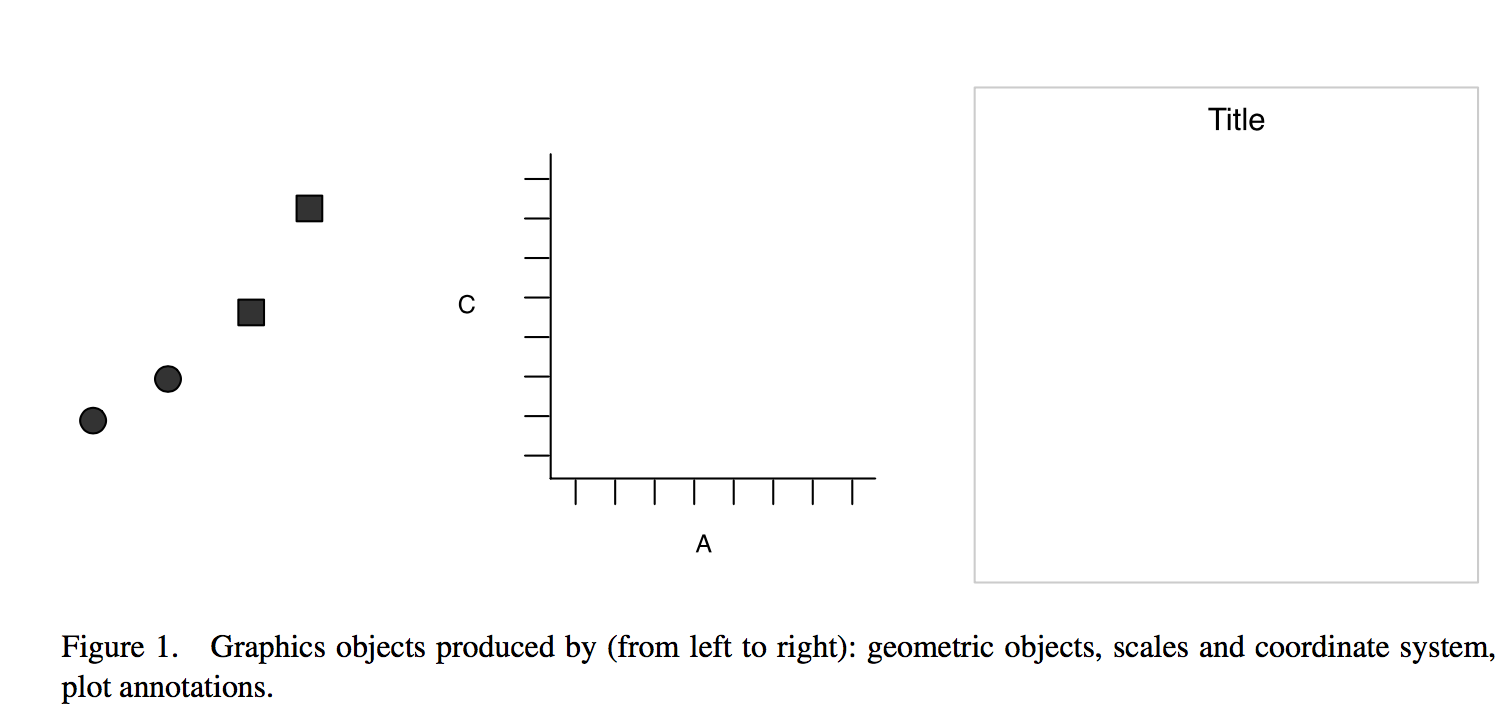source: thinkR

## Simple example

x y shape
25 11 circle
0 0 circle
75 53 square
200 300 square

### 3 layers combined

• aesthetics: x = x, y = y, shape = shape
• geometric object: dot / point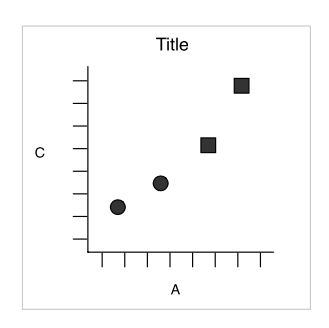### Faceting (treillis / latticing)

What is we want to split circles and squares?

## Faceting

### Split by the shape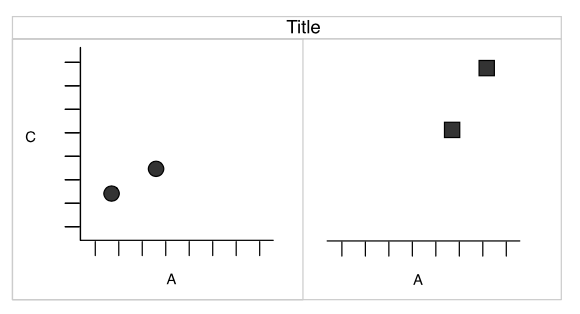### Redundancy

Now, dot shapes and facets give the same information. Shapes could be freed for another meaningful variable

## layers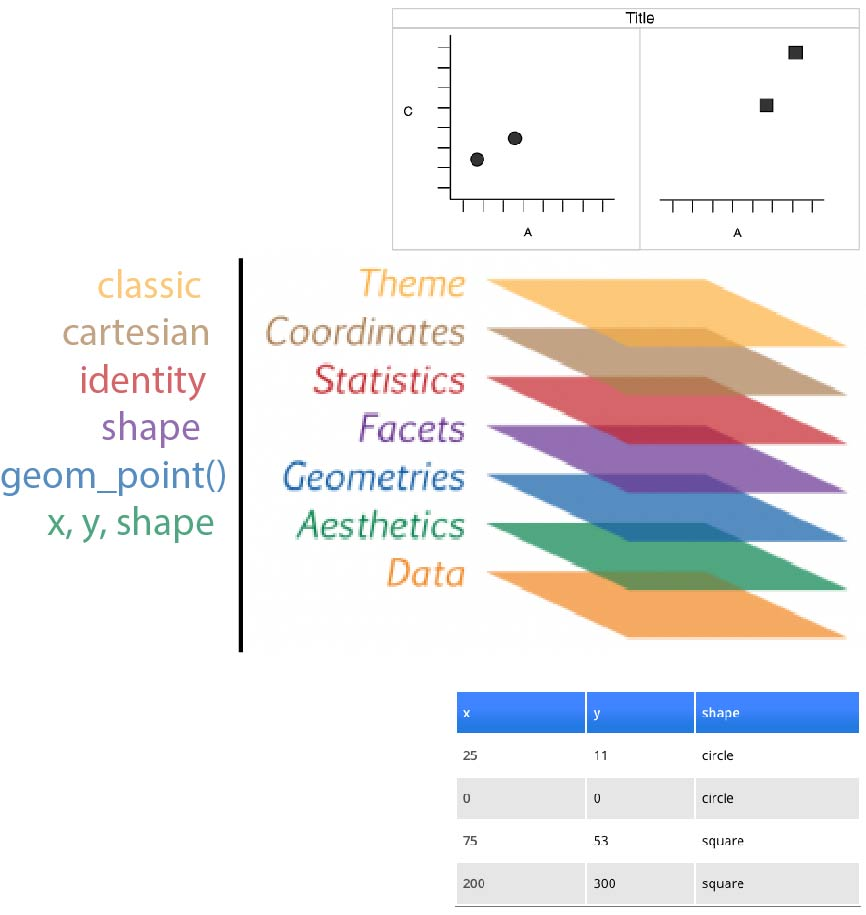## for real

tribble(
~x,   ~y,    ~shape,
25L,  11L,  "circle",
0L,   0L,  "circle",
75L,  53L,  "square",
200L, 300L,  "square"
) %>%
ggplot(aes(x = x, y = y, shape = shape)) +
geom_point(size = 4) +
facet_wrap(~ shape) +
coord_cartesian() +
theme_classic(base_size = 18)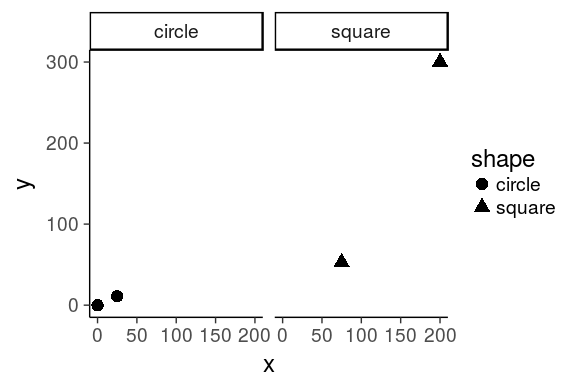### Tip

awesome addin: datapasta, paste as tribbles (transposed tibbles)

by Miles McBrain## Motivation for this layered system

### football example

Data visualisation is not meant just to be seen but to be read, like written text Alberto Cairo

Using the following dataset from the Euro Club Index

library(tidyverse)
oneSeason <- allSeasons %>% filter(year == 2016)
allSeasons
# A tibble: 1,556 x 12
club score  year country     n  rank allRank   atb   atw    eb
<chr> <int> <int>   <chr> <int> <int>   <int> <int> <int> <int>
1     ManUnited  1876  2001     ENG    20     1       6  2031  1831  1927
2     Liverpool  1876  2001     ENG    20     2       5  2020  1826  1918
3         Leeds  1843  2001     ENG    20     3      11  1979  1793  1880
4       Arsenal  1820  2001     ENG    20     4      14  1946  1776  1868
5       Chelsea  1794  2001     ENG    20     5      18  1860  1738  1865
6       Ipswich  1744  2001     ENG    20     6      31  1830  1710  1840
7    Sunderland  1732  2001     ENG    20     7      34  1802  1688  1813
8    AstonVilla  1725  2001     ENG    20     8      42  1775  1667  1796
9     Newcastle  1704  2001     ENG    20     9      46  1765  1663  1792
10 Middlesbrough  1699  2001     ENG    20    10      49  1737  1661  1791
# ... with 1,546 more rows, and 2 more variables: ew <int>, tenth <int>

source John Burn-Murdoch working at the Financial Times

## questions & solutions

### Questions

1. which countries have the best teams?
2. which leagues are the most/least balanced?
3. what is the 'quality gap' between a given pair of leagues?
4. how does the nth best team in league x today compare to its predecessors?
5. how have all of the above changed over time?

### Stat solutions

1. linear comparison
2. distribution of parts within the whole
3. difference in area between two curves
4. value in context
5. evolution of an already detailed pattern over time

### Visual solutions

1. points

2. points on a line

3. ribbon

5. faceted plots

source John Burn-Murdoch working at the Financial Times

## 1. which countries have the best teams in 2016?

oneSeason %>%
ggplot(aes(x = year, y = score, colour = country)) +
geom_point(size = 3) +
scale_x_discrete() +
theme_bw(base_size = 18)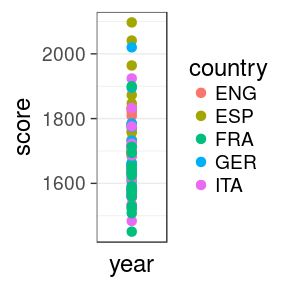• size = 3 increases the size of all dots. Not in aes()
• scale_x_discrete is to force the 1 value on the x axis to be discrete
• theme_bw() is a pre-defined black/white theme, where all fonts are set to size = 18

### Issue

we can't see much. Improve the x mapping

## 1. which countries have the best teams in 2016?

### with rank

oneSeason %>%
ggplot(aes(x = rank, y = score,
colour = country)) +
geom_point(size = 3) +
theme_bw(18)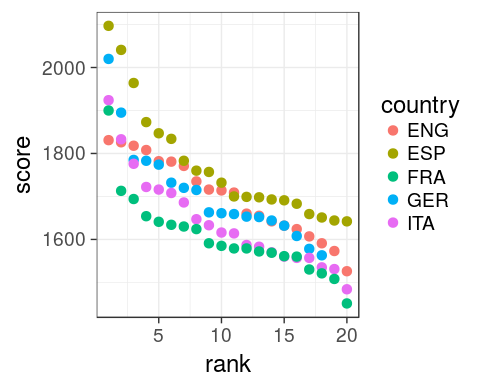• scale_x_discrete is useless now, we have a continuous variable.
• omit base_size = in theme_bw() as it is the first argument.

### Spain

Now obvious that Spain does well, even for low ranking clubs

## 2. which leagues are most/least balanced in 2016?

oneSeason %>%
ggplot(aes(rank, score,
colour = country)) +
geom_line() +
geom_point(size = 3) +
theme_bw(18)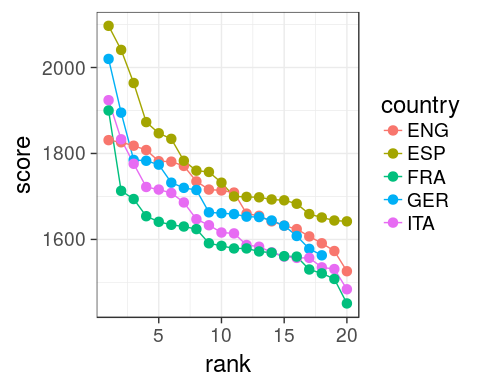• aes() define in ggplot() are passed on all subsequent geom
• x and y could be omitted, better to specify them though.

### Issue

Hard to see differences, ENG seems more coherent

## 2. which leagues are most/least balanced in 2016?

### with range

oneSeason %>%
group_by(country) %>%
summarise(min = min(score),
max = max(score),
range = max - min) %>%
mutate(country = forcats::fct_reorder(country, range)) %>%
ggplot(aes(x = "2016", y = range, fill = country)) +
geom_col(position = "dodge") +
theme_classic(18)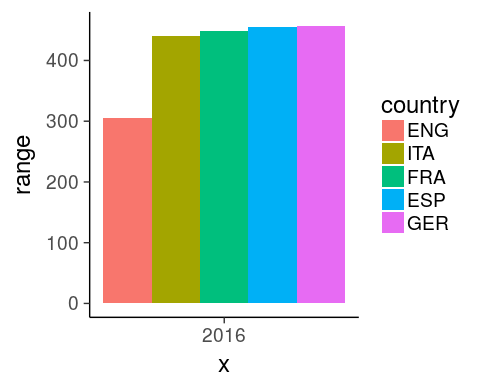### Tip x-axis

force the discretization using 2016 as character

### Tip fill

use dodging to get all bars on the same x index

### Tip fill order

reorder levels based on a numeric variable using fct_reorder

## 3. Quality gap between England and Spain in 2016?

### score diff at each rank

oneSeason %>%
select(score, rank, country) %>%
filter(country %in% c("ENG", "ESP")) %>%
rowwise() %>%
mutate(gap = ESP - ENG,
min = min(ESP, ENG),
max = max(ESP, ENG)) %>%
ggplot(aes(x = rank, fill = gap > 0)) +
geom_rect(aes(xmin = rank - 0.5,
xmax = rank + 0.5,
ymin = min, ymax = max), alpha = 0.8) +
theme_classic(18) +
scale_fill_manual(name = "gap", labels = c("ENG", "ESP"),
values = c("royalblue", "red3")) +
labs(title = "quality gap",
subtitle = "between England and Spain",
caption = "by John Burn-Murdoch")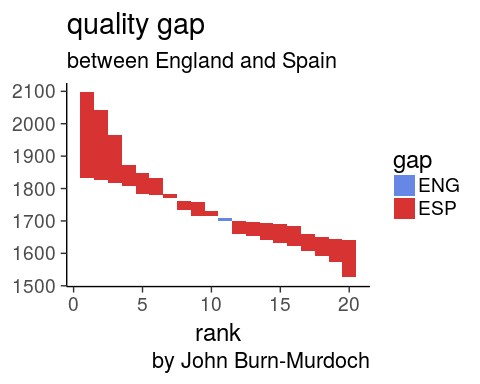• spread so diff is easy to compute between column
• rowwise() mandatory to get the right min and max

### Spain clubs

are performing better at every rank except #11

## 4.How does the nth best English team in 2016 compare to its predecessors?

oneSeason %>%
filter(country == "ENG") %>%
ggplot(aes(x = rank, y = score)) +
geom_ribbon(aes(ymin = atw, ymax = atb),
fill = "royalblue", alpha = 0.5) +
geom_line(size = 1.5, colour = "royalblue") +
geom_point(size = 3, colour = "royalblue") +
theme_bw(18) +
scale_fill_manual(name = "gap", labels = c("ENG", "ESP"),
values = c("royalblue", "red3")) +
labs(title = "Comparison of the nth best \nteam to its predecessors",
subtitle = "in England in 2016",
caption = "by John Burn-Murdoch")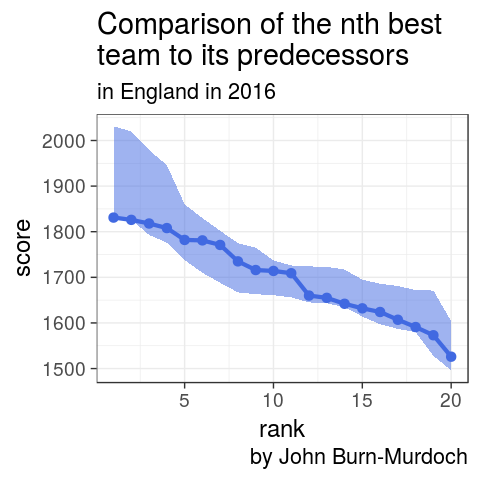## 5. How to visualise over time?

### facet: best country

allSeasons %>%
ggplot(aes(rank, score,
colour = country)) +
geom_line() +
#geom_point(size = 1.5) +
theme_classic(18) +
facet_wrap(~ year)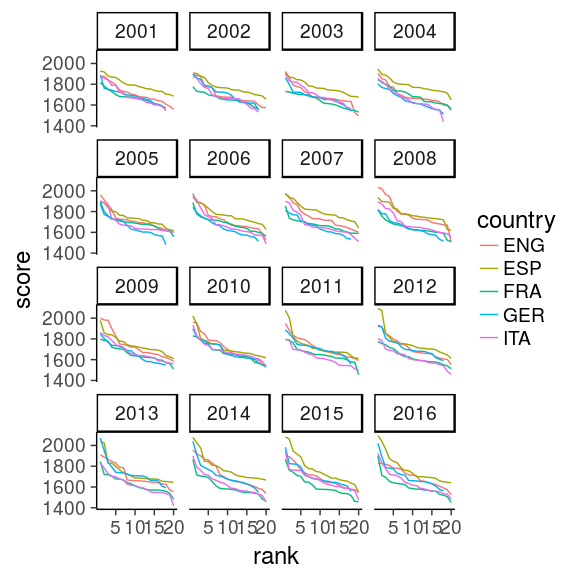## 5. How to visualise over time?

### facet: gap code

allSeasons %>%
select(score, year, rank, country) %>%
filter(country %in% c("ENG", "ESP")) %>%
rowwise() %>%
mutate(gap = ESP - ENG,
min = min(ESP, ENG),
max = max(ESP, ENG)) %>%
ggplot(aes(x = rank, fill = gap > 0)) +
geom_rect(aes(xmin = rank - 0.5,
xmax = rank + 0.5,
ymin = min, ymax = max), alpha = 0.8) +
theme_classic(18) +
scale_fill_manual(name = "gap", labels = c("ENG", "ESP"),
values = c("royalblue", "red3")) +
labs(title = "quality gap",
subtitle = "between England and Spain",
caption = "by John Burn-Murdoch") +
facet_wrap(~ year)

With tidy data, add only the facet layer to get all panels

## 5. How to visualise over time?

### facet: gap plot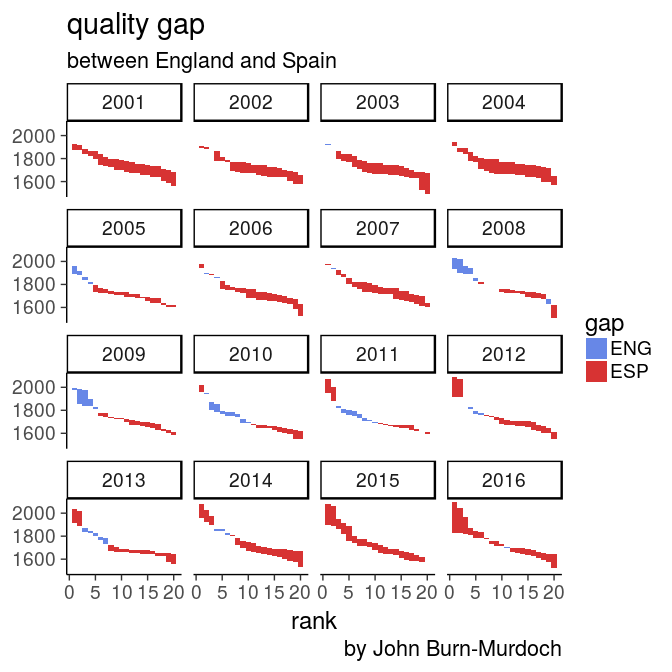## iris dataset

rstudio cheatsheet

iris <- as_tibble(iris)
iris
# A tibble: 150 x 5
Sepal.Length Sepal.Width Petal.Length Petal.Width Species
<dbl>       <dbl>        <dbl>       <dbl>  <fctr>
1          5.1         3.5          1.4         0.2  setosa
2          4.9         3.0          1.4         0.2  setosa
3          4.7         3.2          1.3         0.2  setosa
4          4.6         3.1          1.5         0.2  setosa
5          5.0         3.6          1.4         0.2  setosa
6          5.4         3.9          1.7         0.4  setosa
7          4.6         3.4          1.4         0.3  setosa
8          5.0         3.4          1.5         0.2  setosa
9          4.4         2.9          1.4         0.2  setosa
10          4.9         3.1          1.5         0.1  setosa
# ... with 140 more rows

### global definition of the iris dataset

as a tibble to avoid printing all 150 rows

### change default theme

I set for this course the following to avoid the grey background and print bigger text

ggplot2::theme_set(ggplot2::theme_bw(18))

iris %>%
ggplot() +
geom_point(aes(x = Petal.Width, y = Petal.Length))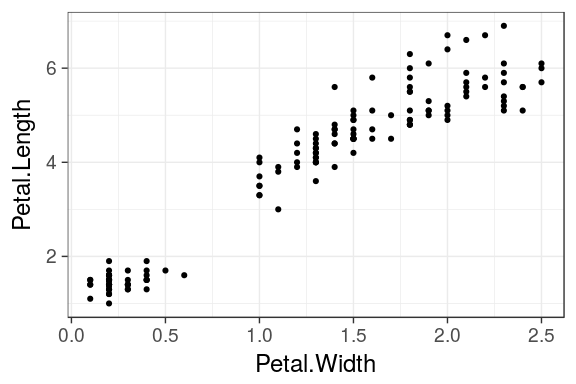## geometric objects

### geom_point()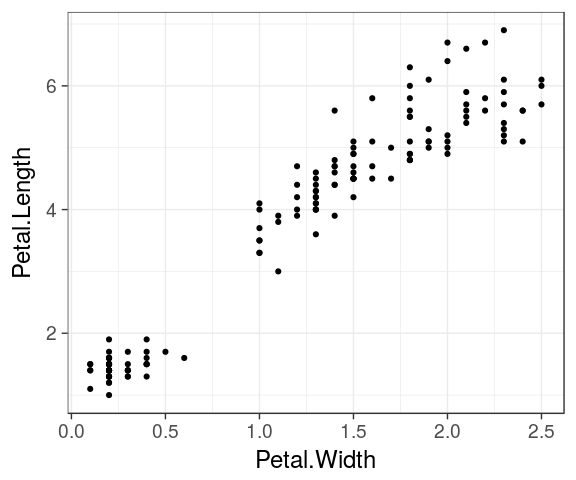### geom_line()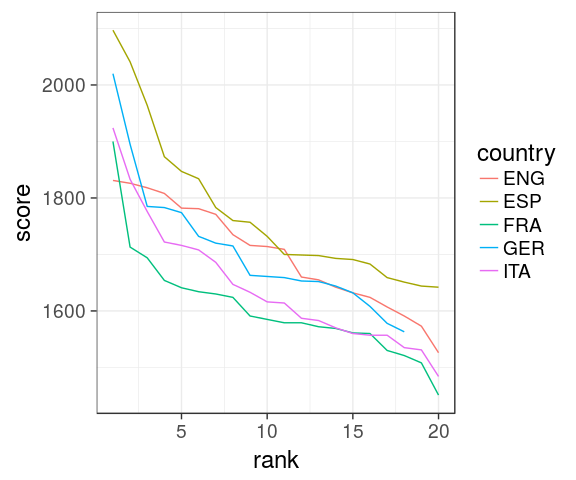### geom_bar()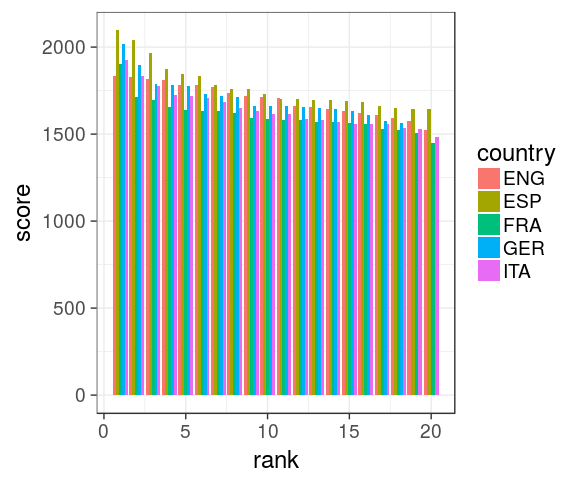### geom_boxplot()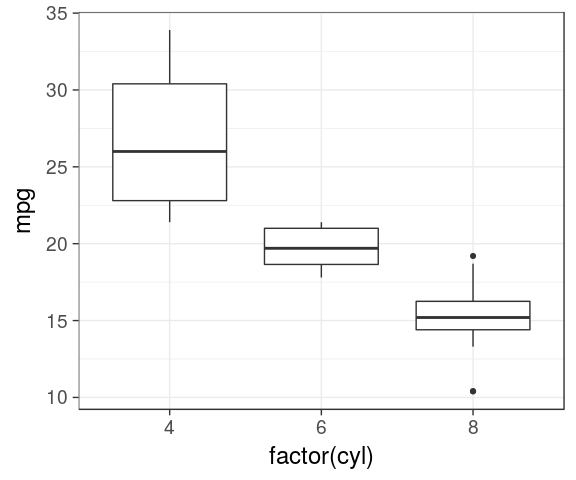### geom_histogram()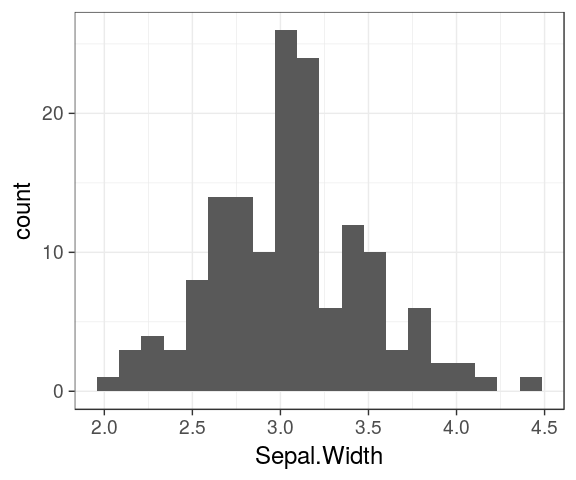### geom_density()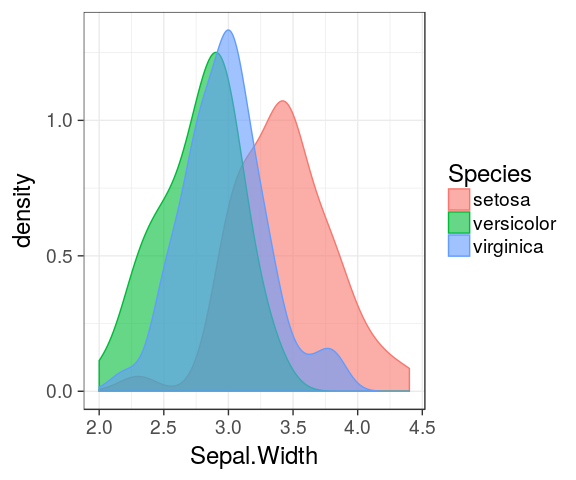## Mapping aesthetics

aesthetics map the columns of a data.frame/tibble to the variable each ggplot2 geom is expecting.

For example geom_point() requires at least the x and y coordinates for each point.

ggplot(iris) +
geom_point(aes(x = Petal.Width, y = Petal.Length))
Sepal.Length Sepal.Width Petal.Length Petal.Width Species
5.1 3.5 1.4 0.2 setosa
4.9 3.0 1.4 0.2 setosa
4.7 3.2 1.3 0.2 setosa

## Unmapped paramaters

Additional arguments such as the colour, the transparency (alpha) or the size.

ggplot(iris) +
geom_point(aes(x = Petal.Width,
y = Petal.Length),
colour = "blue", alpha = 0.6,
size = 3)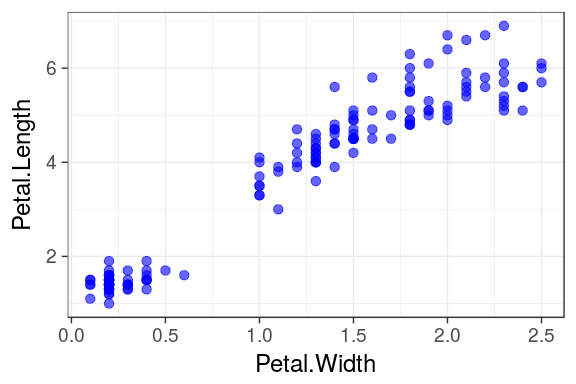### Important

see that paramaters define outside the aesthetics aes() are applied to all data

## Mapping aesthetics

### colour

colour, alpha or size can also be mapped to a column in the data frame.

For example: We can attribute a different color to each species:

ggplot(iris) +
geom_point(aes(x = Petal.Width,
y = Petal.Length,
colour = Species),
alpha = 0.6, size = 3)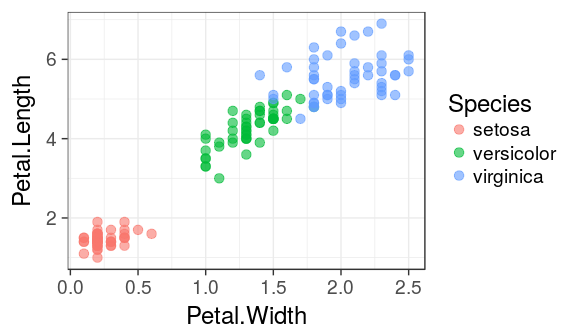### Important

Note that the colour argument now is inside aes() and must refer to a column in the dataframe.

## Mapping aesthetics

### shape

ggplot(iris) +
geom_point(aes(x = Petal.Width, y = Petal.Length, shape = Species, colour = Species),
alpha = 0.6, size = 3)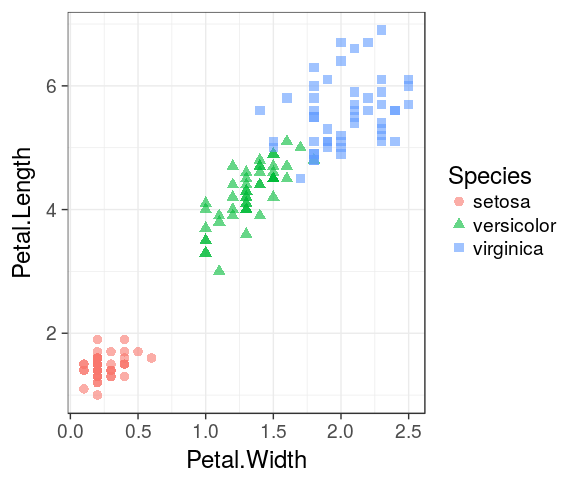## Labels

It is easy to adjust axis labels and the title

ggplot(iris) +
geom_point(aes(x = Petal.Width,
y = Petal.Length,
colour = Species),
alpha = 0.6, size = 3) +
labs(x = "Width",
y = "Length",
colour = "flower",
title = "Iris",
subtitle = "petal measures",
caption = "Fisher, R. A. (1936)")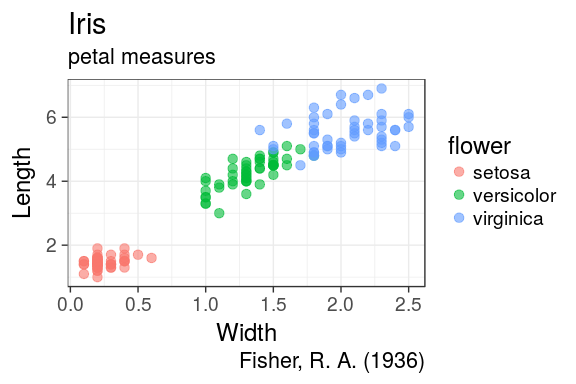## Histograms

ggplot(iris) +
geom_histogram(aes(x = Petal.Length,
fill = Species),
alpha = 0.6) 
stat_bin() using bins = 30. Pick better value with binwidth.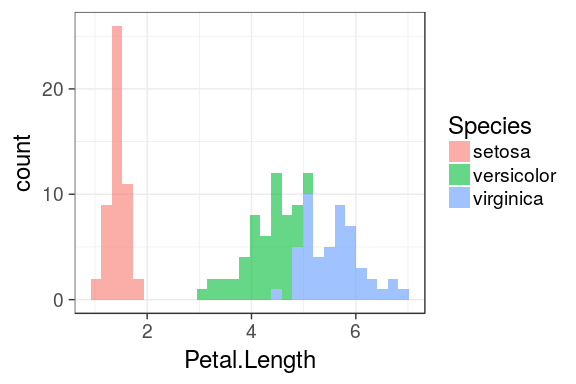## Density plot

The density is the count divided by the total number of occurences.

ggplot(iris) +
geom_density(aes(x = Petal.Length,
fill = Species),
alpha = 0.6)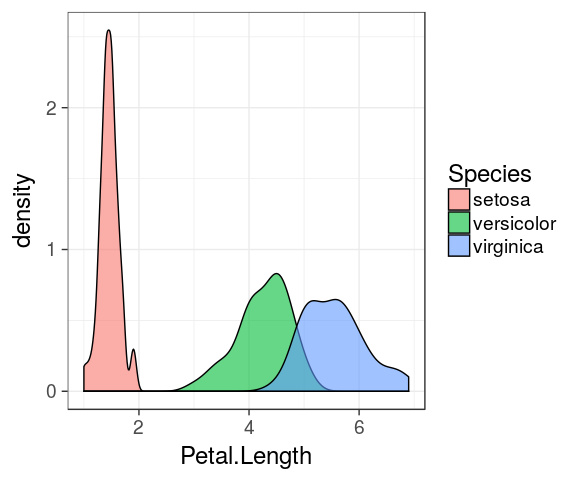## Overlaying plots

### Density plot and histogram

ggplot(iris) +
geom_histogram(aes(x = Petal.Length, y = ..density..), fill = "darkgrey", binwidth = 0.1) +
geom_density(aes(x = Petal.Length, fill = Species, colour = Species), alpha = 0.4) +
theme_classic()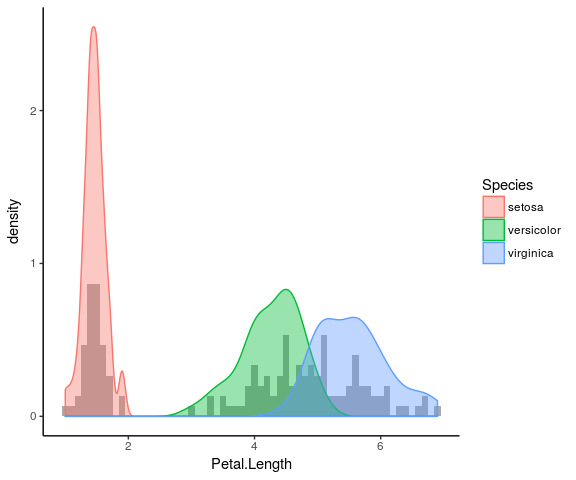## Stat functions

### transform data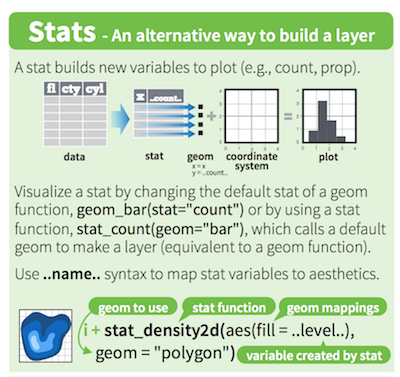• variables surrounded by two pair of dots (..variable..) are intermediate values calculated by ggplot2 using stat functions
• geom uses a stat function to transform the data:
• geom_histogram() uses stat_bin() to divide the data into bins and count the number of observations in each bin.
• stat_bin() computes for example: ..count.., ..density.., ..ncount.. and ..ndensity.. (see ?stat_bin())
• stat variable for density plot: ..density..
• stat_identity is used for scatter plots or geom_col(), no transformation

### 1D

• stat_count
• stat_bin

### 2D

• stat_density_2d
• stat_bin_2d
• stat_ellipse

### 3D

• stat_contour
• stat_summary_hex

source: ggplot2 cheatsheet by Rstudio

## Barcharts

### Categorical variables

• By default, geom_bar() counts the number of occurences for each values of a categorical variable.
• geom_bar() uses stat_count() to compute these values (creating a new count column)
ggplot(iris) +
geom_bar(aes(x = Species))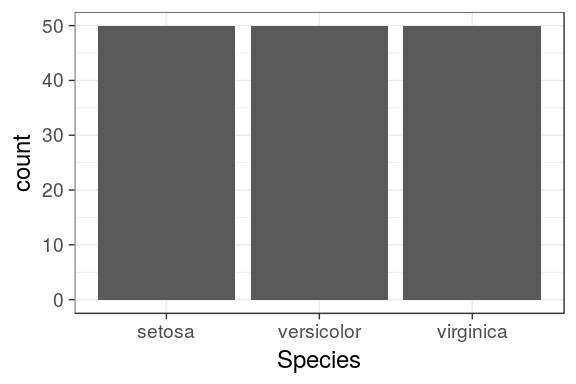# or: geom_bar(aes(x = Species, y = ..count..))

## Barcharts

### Categorical variables

• To map a continous variable on the y axis, we need to override the default call to stat_count()
• stat = "identity" will force geom_bar() to use stat_identity() instead (leaving the original data unchanged)
• Petal.Length and not ..Petal.Length.. as it is not "new" and is already present in the original data frame
ggplot(iris) +
geom_bar(aes(x = Species, y = Petal.Length), stat = "identity")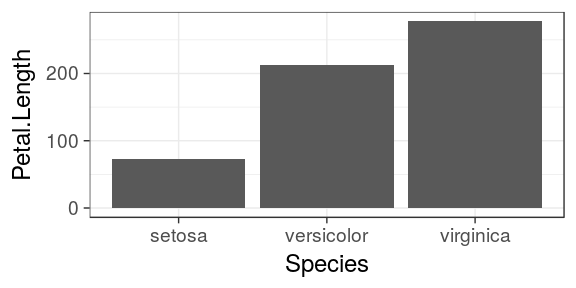### Update v2.1

since version 2.1, thanks to Bob Rudis, geom_col does require a y variable

### geom_col

ggplot(iris) +
geom_col(aes(x = Species, y = Petal.Length))

## Stacked barchart

### categorical variables

mtcars %>%
ggplot() +
geom_bar(aes(x = factor(cyl),
fill = factor(gear)))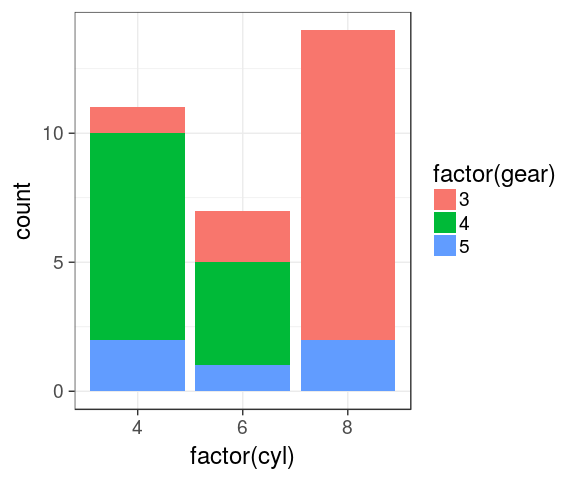## Dodged barchart (side by side)

### categorical variables

mtcars %>%
mutate(cyl = factor(cyl),
gear = factor(gear)) %>%
complete(cyl, gear) %>%
ggplot() +
geom_bar(aes(x = cyl,
fill = gear),
position = "dodge")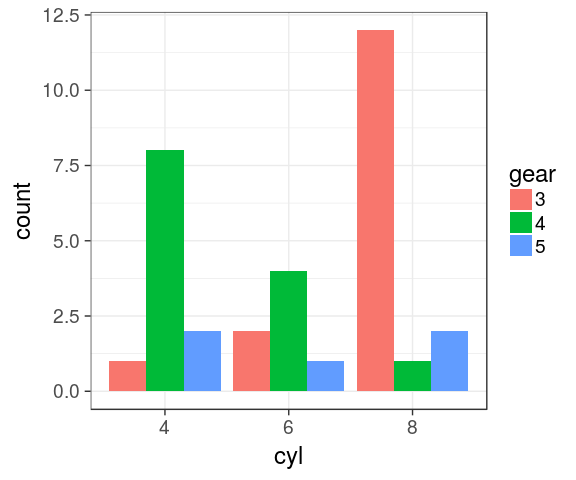### Complete

the combination gear 4 / cyl 8 is missing. Using tidyr::complete() to avoid bars with different widths.

## Stacked barchart for proportions

### categorical variables

mtcars %>%
mutate(cyl = factor(cyl),
gear = factor(gear)) %>%
complete(cyl, gear) %>%
ggplot() +
geom_bar(aes(x = cyl,
fill = gear),
position = "fill")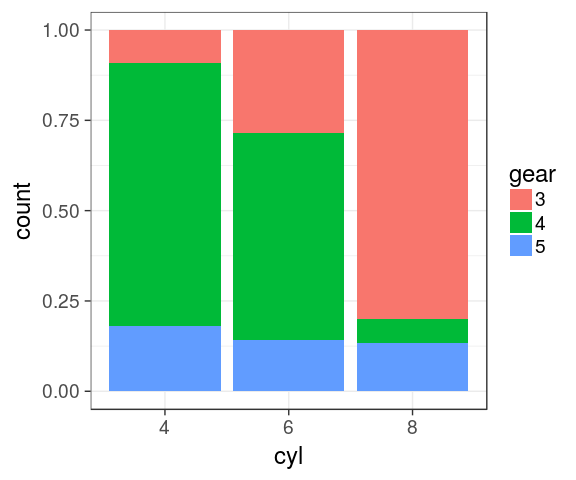## Stacked barchart for proportions

### pie charts

We can easily switch to polar coordinates:

mtcars %>%
mutate(cyl = factor(cyl),
gear = factor(gear)) %>%
complete(cyl, gear) %>%
ggplot() +
geom_bar(aes(x = cyl,
fill = gear),
position = "fill") +
coord_polar()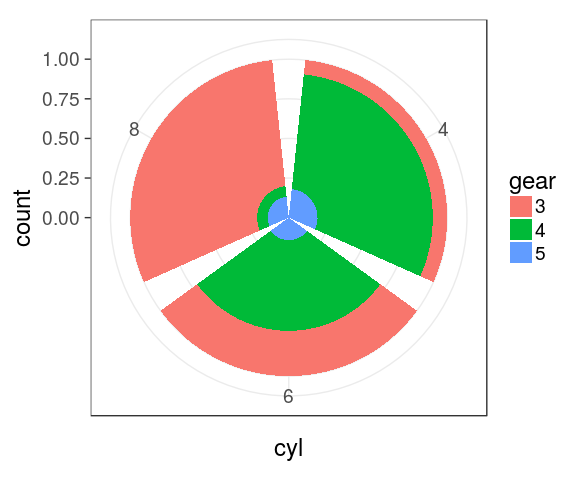## Boxplot

### IQR, median

ggplot(mtcars) +
geom_boxplot(aes(x = factor(cyl),
y = mpg))## Boxplot

### dodge by default

ggplot(mtcars) +
geom_boxplot(aes(x = factor(cyl),
y = mpg,
fill = factor(am)))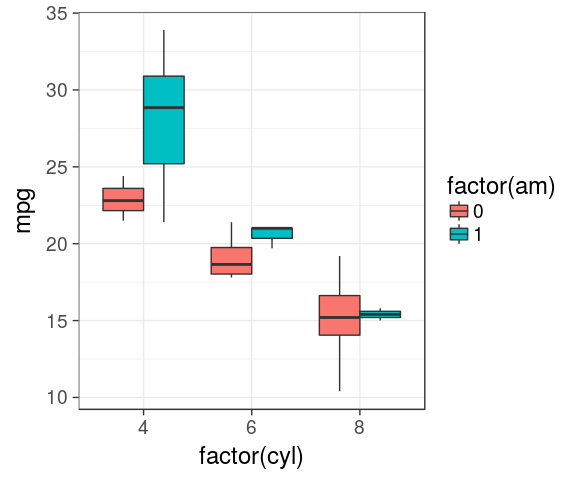## Custom colors

• It is possible to manually adjust the colors using scale_fill_manual() and scale_color_manual()
• Is not very handy as you must provide as much colours as groups
ggplot(mtcars) +
geom_boxplot(aes(x = factor(cyl),
y = mpg,
fill = factor(am),
color = factor(am))) +
scale_fill_manual(values = c("red", "lightblue")) +
scale_color_manual(values = c("purple", "blue"))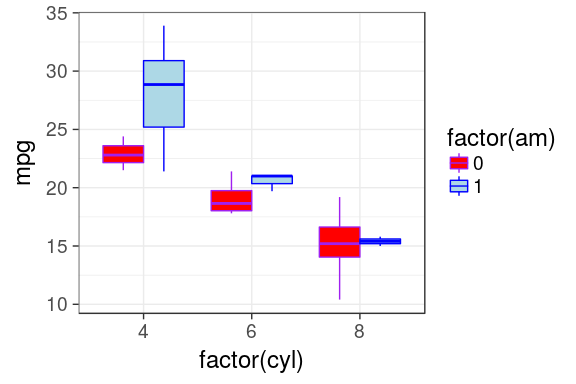## Predefined color palettes

library(RColorBrewer)
display.brewer.all()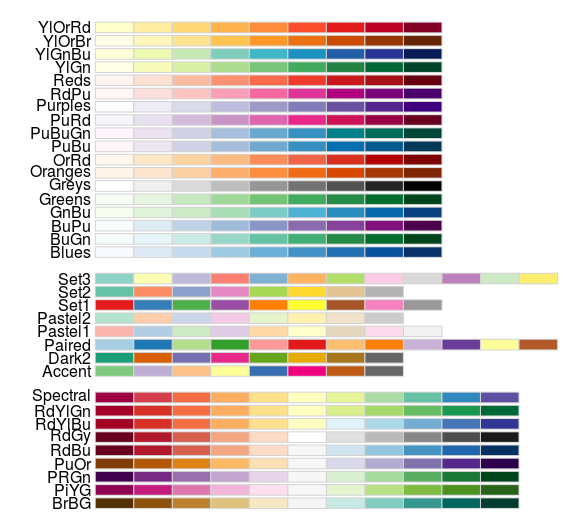## Custom colors

### using brewer

ggplot(mtcars) +
geom_boxplot(aes(x = factor(cyl),
y = mpg,
fill = factor(am),
colour = factor(am))) +
scale_fill_brewer(palette = "Pastel2") +
scale_colour_brewer(palette = "Set1")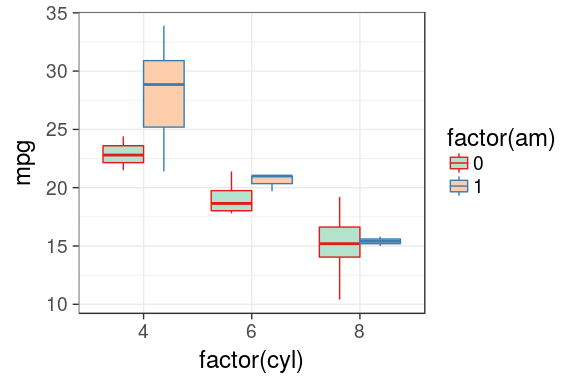### default

mtcars %>%
ggplot(aes(x = wt,
y = mpg,
colour = hp)) +
geom_point(size = 3)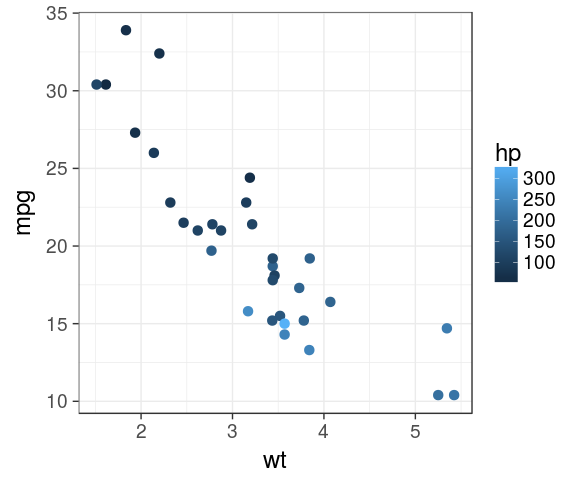### viridis

mtcars %>%
ggplot(aes(x = wt,
y = mpg,
colour = hp)) +
geom_point(size = 3) +
viridis::scale_colour_viridis()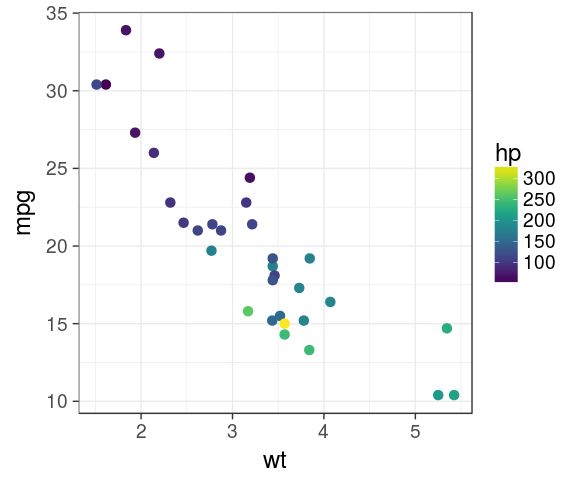viridis is color blind friendly and nice in b&w

### 4 different scales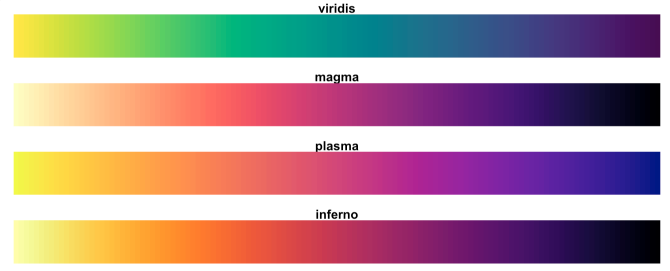## aesthetic trick

Actually, one can use a plain character inside aes(), will be used to build the legend. Useful for few layers when lazy enough to create the variable in the dataframe.

set.seed(123)
dens <- tibble(x = c(rnorm(500),
rnorm(200, 3, 3)))
ggplot(dens) +
geom_line(aes(x), stat = "density") +
geom_vline(aes(xintercept = mean(x),
colour = "mean"),
size = 1.1) +
geom_vline(aes(xintercept = median(x),
colour = "median"),
size = 1.1) -> p
p## the data argument

### each layer can get its own

dens_mode <- tibble(mode = density(dens$x)$x[which.max(density(dens$x)$y)])
p + geom_vline(data = dens_mode,
aes(xintercept = mode, colour = "mode"), size = 1.1) +
theme(legend.position = "top") +
scale_colour_hue(name = NULL) # could be: labs(colour = NULL)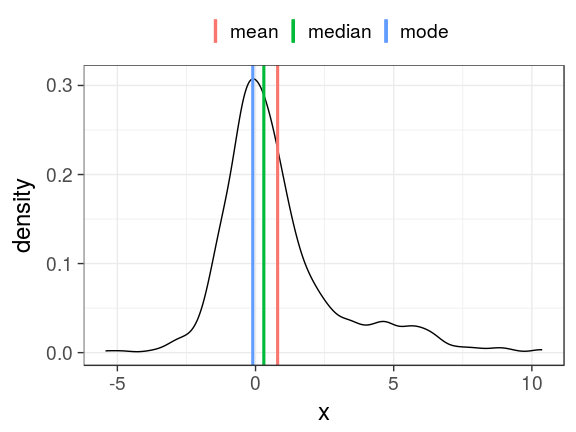## Facets

### facet_wrap()

the easiest way to create facet is to provide facet_wrap() with a column name

ggplot(mtcars) +
geom_point(aes(x = wt, y = mpg)) +
facet_wrap(~ cyl)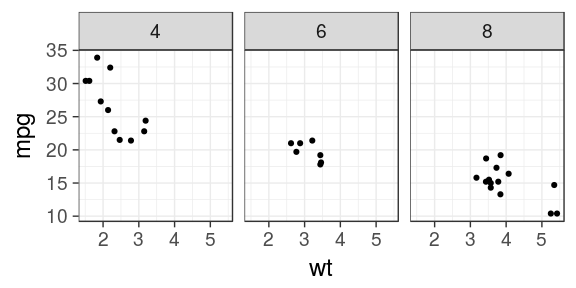ggplot(mtcars) +
geom_point(aes(x = wt, y = mpg)) +
facet_wrap(~ cyl, ncol = 2)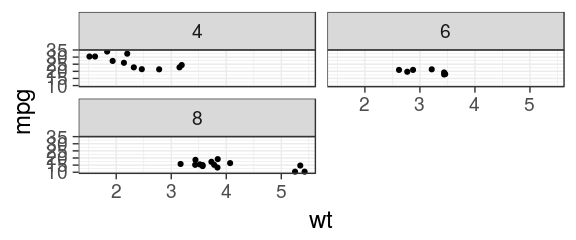## Facets

### free scales

ggplot(mtcars) +
geom_point(aes(x = wt, y = mpg)) +
facet_wrap(~ cyl, scales = "free_x")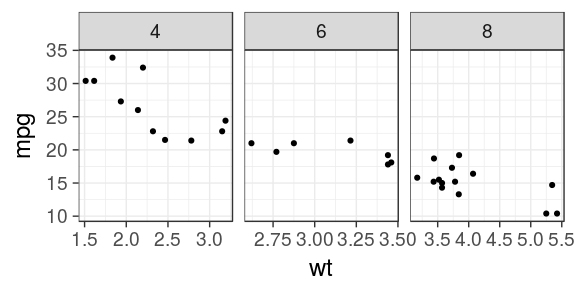ggplot(mtcars) +
geom_point(aes(x = wt, y = mpg)) +
facet_wrap(~ cyl, scales = "free")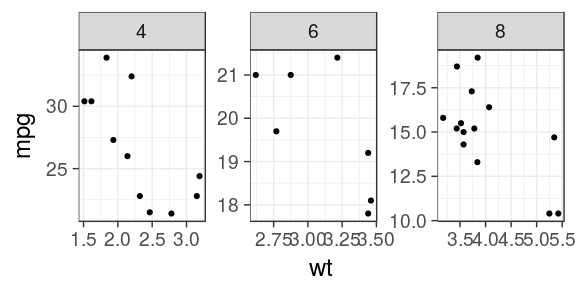## Facets

### Specify a formula

the rows on the left and columns on the right separated by a tilde ~ (i.e by)

ggplot(mtcars) +
geom_point(aes(x = wt, y = mpg)) +
facet_grid(am ~ cyl)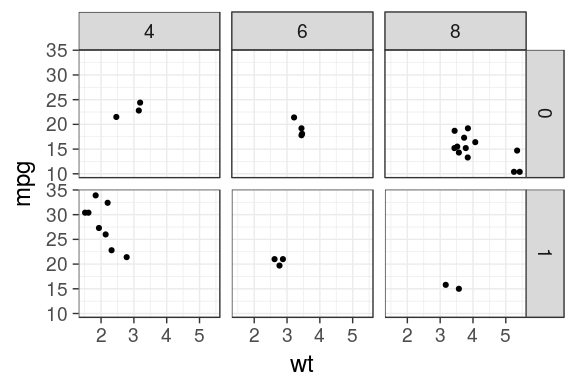## Facets

### Specify one row/column

A dot (.) specifies that no faceting should be performed. Mimic facet_wrap()

ggplot(mtcars) +
geom_point(aes(x = wt, y = mpg)) +
facet_grid(. ~ cyl)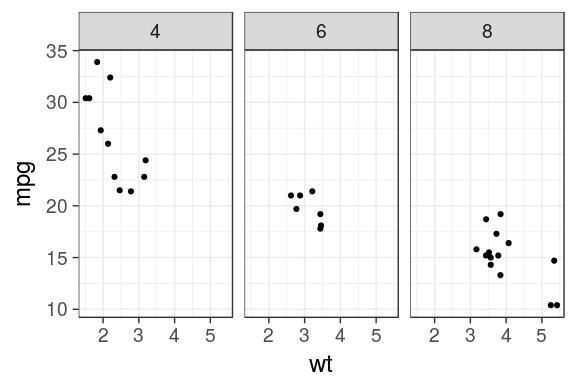## Facets

### labeller

Add the column names with labeller

ggplot(mtcars) +
geom_point(aes(x = wt, y = mpg)) +
facet_grid(am ~ cyl,
labeller = label_both)## Exporting

### right panel

• Using the Export button in the Plots panel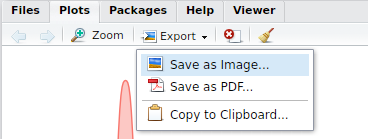### Rmarkdown reports

• If needed, adjust the chunk options:
• size: fig.height, fig.width
• ratio: fig.asp
• others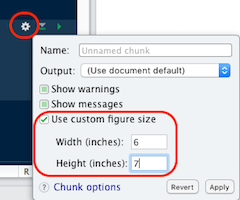### ggsave

• save the ggplot object, 2nd argument
• guess the type of graphics by the extension
ggsave("aes_trick.png", p,
width = 60, height = 30, units = "mm")
ggsave("aes_trick.pdf", p,
width = 50, height = 50, units = "mm")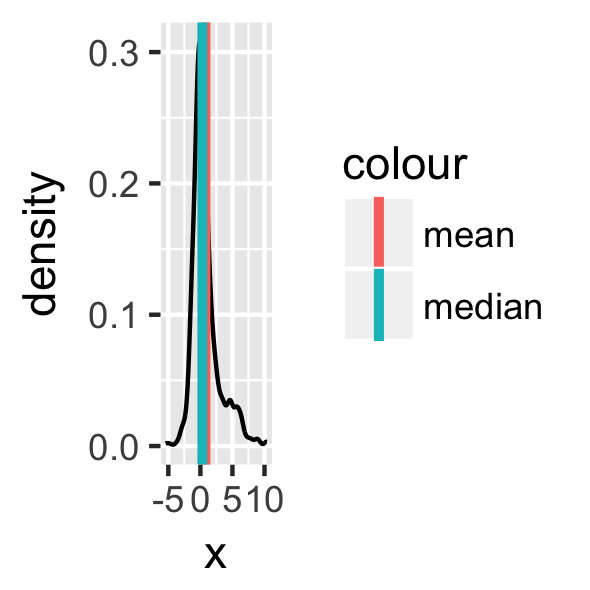## Extensions

ggplot2 introduced the possibility for the community to contribute and create extensions.

They are referenced on a dedicated site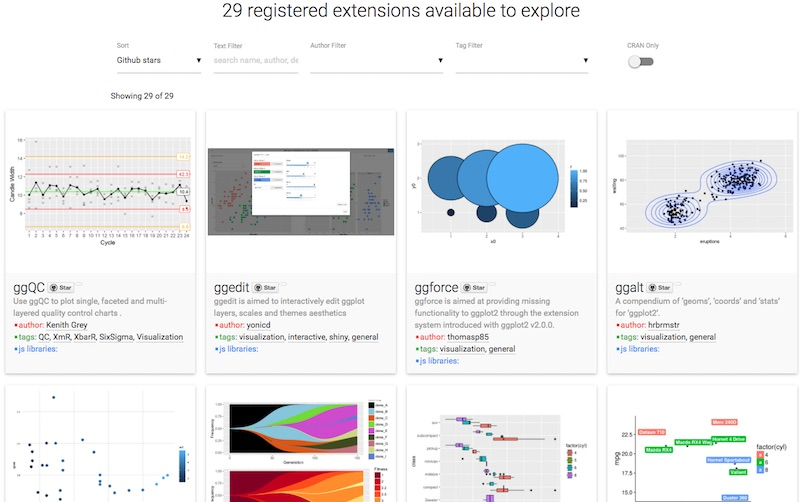### Anscombe ** 10

never trust summary statistics alone; always visualize your data Alberto Cairosource: Justin Matejka, George Fitzmaurice Same Stats, Different Graphs…

## Missing features

### geoms list here

• geom_tile() heatmap
• geom_bind2() 2D binning
• geom_abline() slope

### stats list here

• stat_ellipse()
• stat_summary() easy mean 95CI etc.
• geom_smooth() linear/splines/non linear

### plot on multi-pages

• ggforce::facet_grid_paginate() facets
• gridExtra::marrangeGrob() plots

### positions list here

• position_jitter() random shift

### coordinate / transform

• coord_cartesian() for zooming in
• coord_flip() exchanges x & y
• scale_x_log10() and y
• scale_x_sqrt() and y

### customise theme elements

• legend & guide tweaks
• major/minor grids
• font, faces
• margins
• labels & ticks
• strip positions

### programming

• aes_string() for plotting inside function